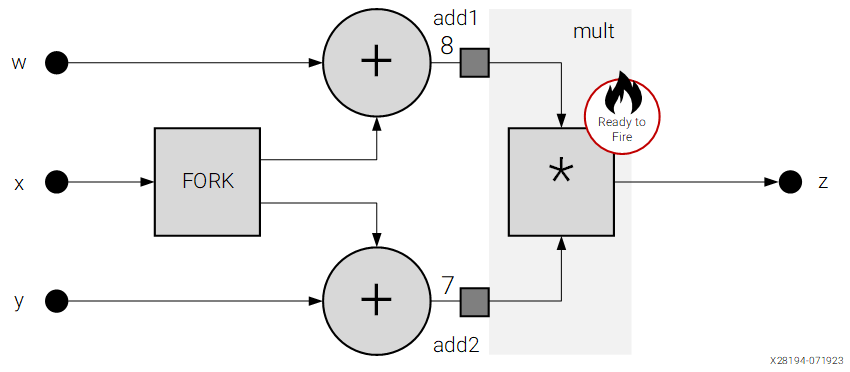# Data Flow Graph

## AI Engine Programming: A Kahn Process Network Evolution (WP552)

Document ID
WP552
Release Date
2023-07-20
Revision
1.0 English

The data flow graph indicates the processing sequence (or precedence), parallelism, and data dependence. The following figure shows a representation of a data flow graph that has inputs that are processed to generate outputs. The three inputs are w, x, and y. In the figure, the inputs w and y are going to separate nodes.

Figure 1. Data Flow Graph Representation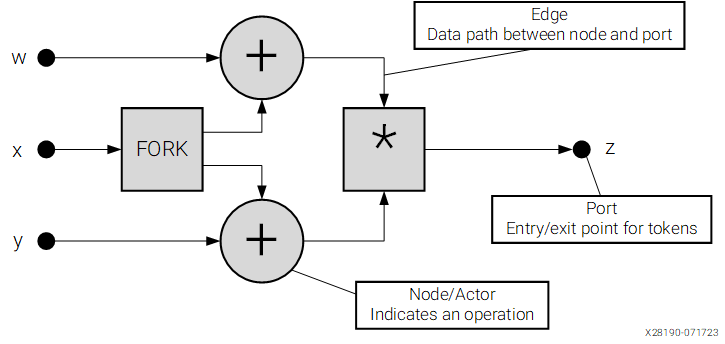Edges indicate the path that the data takes to or from actors or ports. Ports indicate the points at which tokens enter or leave actors or the graph. The data flow graph shows the processing sequence, parallelism, and data dependence.

In the following figure, the fork replicates the input token across multiple outputs. The output of the nodes (edges) is implemented as FIFO buffers.

Figure 2. Fork Replicates the Input Token Across Multiple Outputs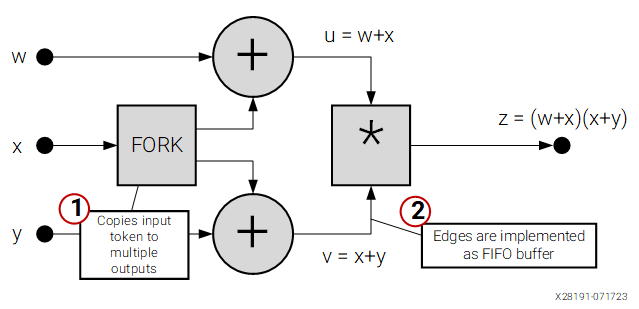Nodes (or actors) fire only when a single token is present on every input to the node. If at least one input is missing a token, the node is blocked. Each token is removed from the edge of each input after firing the node.

In the following figure, the values for w, x, and y are 3, 5, and 2, respectively. The x value is passed to the fork. The fork is ready to fire, while the nodes add1, add2, and mult are waiting for all inputs.

Figure 3. Input Tokens are Available for Fork (Node/Actor) - Ready to Fire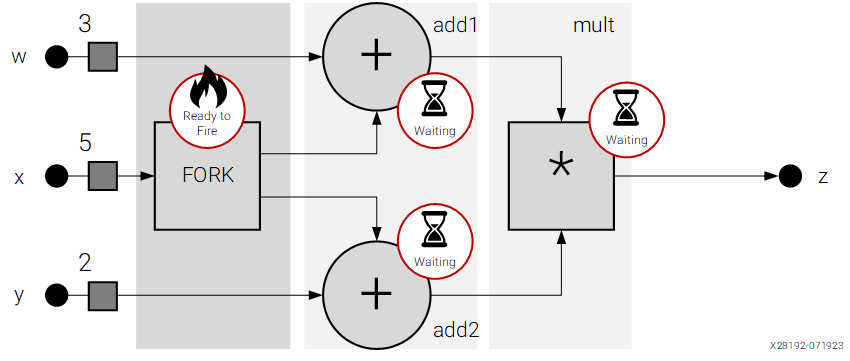In the following figure, the x value 5 has been copied to nodes add1 and add2. The w value 3 and y value 2 are passed to nodes add1 and add2, respectively, from the input nodes. The node mult waits for its input tokens until add1 and add2 are both ready to fire and provide them. The output of node add1 is 8 (that is, 3 + 5). The output of node add2 is 7 (that is, 2 + 5). These output edges are implemented as FIFOs.

Figure 4. Input Tokens are Available for Add1 and Add2 (Node/Actor) - Ready to Fire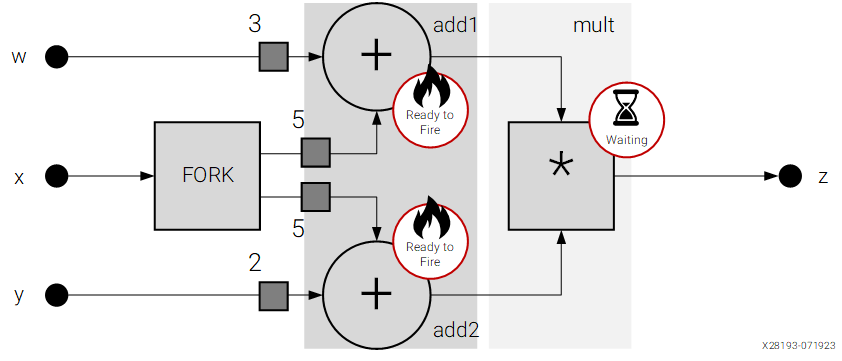In the following figure, the output tokens from add1 and add2 arrive at node mult, which then performs the multiplication and provides the output as 56 (that is, 8×7). This output then goes to port z.

Figure 5. Input Tokens are Available for Mult (Node/Actor) - Ready to Fire